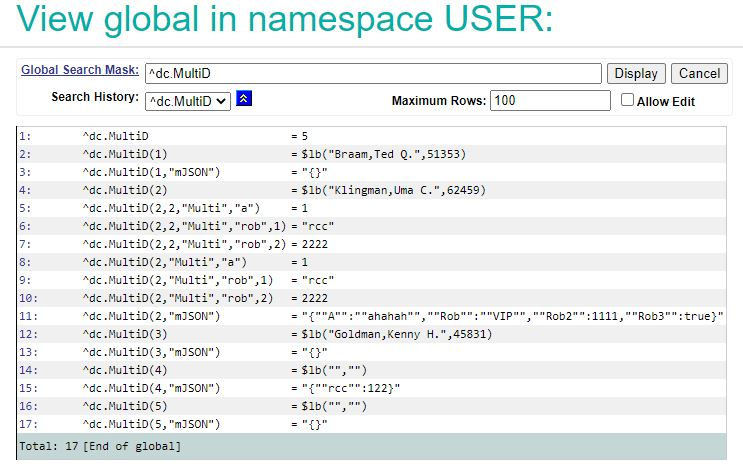﻿ GlobalToJSON-Compact | InterSystems Developer Community | Globals|JSON
Article
Robert Cemper · Jan 24, 2022 1m read

# GlobalToJSON-Compact

I have created a package that offers a utility to load a Global into JSON object and reverse
to create a Global from this type of JSON object. Compact refers to the structure created.
Globals nodes are included with data for a fast data load.
But also the related code is quite compact.

The export of this GlobalGenerates a JSON object that covers all Global nodes

``````USER>write \$\$Do^ZPretty(json)
{
"gbl":[
"^dc.MultiD=5",
"^dc.MultiD(1)=\$lb(\"Braam,Ted Q.\",51353)",
"^dc.MultiD(1,\"mJSON\")=\"{}\"",
"^dc.MultiD(2)=\$lb(\"Klingman,Uma C.\",62459)",
"^dc.MultiD(2,2,\"Multi\",\"a\")=1",
"^dc.MultiD(2,2,\"Multi\",\"rob\",1)=\"rcc\"",
"^dc.MultiD(2,2,\"Multi\",\"rob\",2)=2222",
"^dc.MultiD(2,\"Multi\",\"a\")=1",
"^dc.MultiD(2,\"Multi\",\"rob\",1)=\"rcc\"",
"^dc.MultiD(2,\"Multi\",\"rob\",2)=2222",
"^dc.MultiD(2,\"mJSON\")=\"{\"\"A\"\":\"\"ahahah\"\",\"\"Rob\"\":\"\"VIP\"\",\"\"Rob2\"\":1111,\"\"Rob3\"\":true}\"",
"^dc.MultiD(3)=\$lb(\"Goldman,Kenny H.\",45831)",
"^dc.MultiD(3,\"mJSON\")=\"{}\"",
"^dc.MultiD(4)=\$lb(\"\",\"\")",
"^dc.MultiD(4,\"mJSON\")=\"{\"\"rcc\"\":122}\"",
"^dc.MultiD(5)=\$lb(\"\",\"\")",
"^dc.MultiD(5,\"mJSON\")=\"{}\""
]
}
USER>``````

And the related Loader creates exactly the same Global

Online Demo Terminal
Online Demo SMP

Video

GitHub

5
0 248
Discussion (3)1

I have added a new version  for the GLOBALS contest
To take care of large Globals that may break your available memory.
The JSON Object is exported to a file. and there is also the related loader

### New Version 0.1.2

``````USER>write ##class(dc.GblToJSON.CX).export("^dc.MultiD")
File gbl.json created
``````

And the related loader creates the Global

``````USER>write ##class(dc.GblToJSON.CX).import()
``````

It is of course also available in the Online Demo facility.

And to see the generated file there is a show() method

``````USER>write ##class(dc.GblToJSON.CX).show()
{"gbl":[
"^dc.MultiD=5",
"^dc.MultiD(1)=\$lb(\"Braam,Ted Q.\",51353)",
"^dc.MultiD(1,\"mJSON\")=\"{}\"",
"^dc.MultiD(2)=\$lb(\"Klingman,Uma C.\",62459)",
"^dc.MultiD(2,2,\"Multi\",\"a\")=1",
"^dc.MultiD(2,2,\"Multi\",\"rob\",1)=\"rcc\"",
"^dc.MultiD(2,2,\"Multi\",\"rob\",2)=2222",
"^dc.MultiD(2,\"Multi\",\"a\")=1",
"^dc.MultiD(2,\"Multi\",\"rob\",1)=\"rcc\"",
"^dc.MultiD(2,\"Multi\",\"rob\",2)=2222",
"^dc.MultiD(2,\"mJSON\")=\"{\"\"A\"\":\"\"ahahah\"\",\"\"Rob\"\":\"\"VIP\"\",\"\"Rob2\"\":1111,\"\"Rob3\"\":true}\"",
"^dc.MultiD(3)=\$lb(\"Goldman,Kenny H.\",45831)",
"^dc.MultiD(3,\"mJSON\")=\"{}\"",
"^dc.MultiD(4)=\$lb(\"\",\"\")",
"^dc.MultiD(4,\"mJSON\")=\"{\"\"rcc\"\":122}\"",
"^dc.MultiD(5)=\$lb(\"\",\"\")",
"^dc.MultiD(5,\"mJSON\")=\"{}\""
]}
***** gbl.json *****
USER>
``````